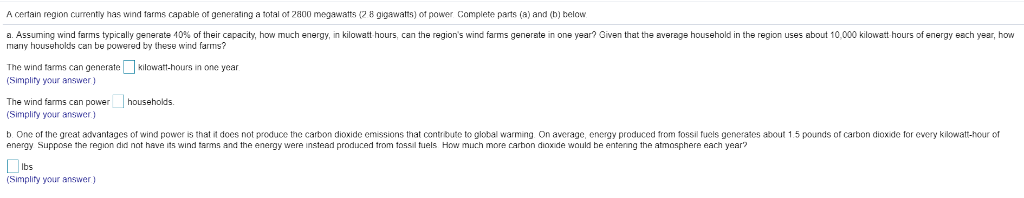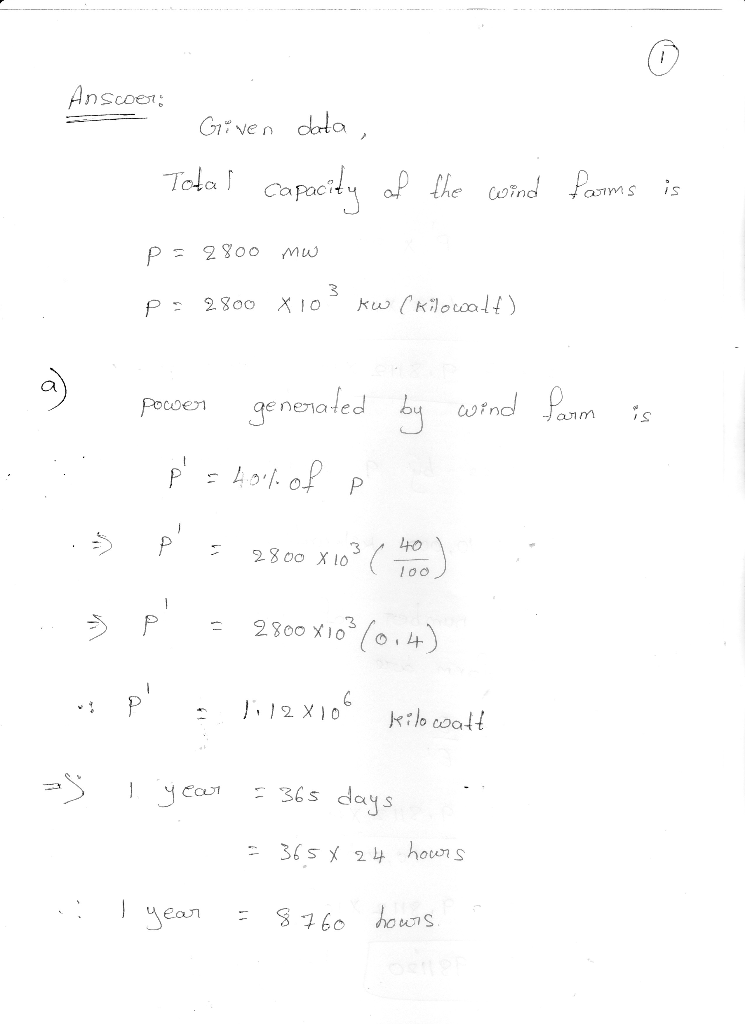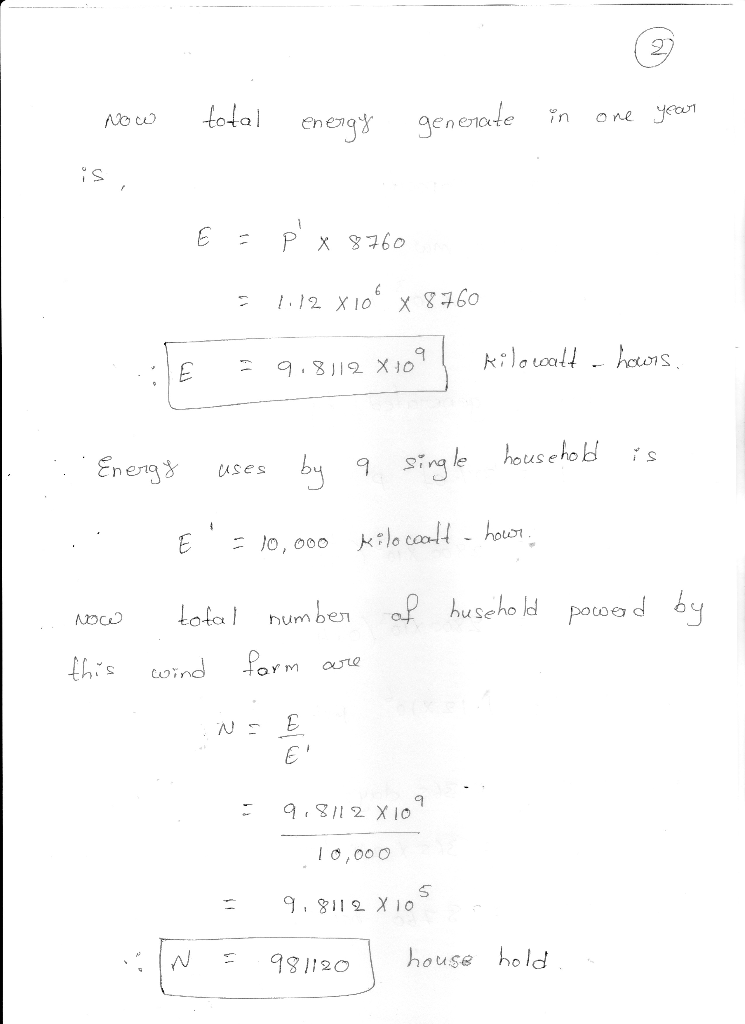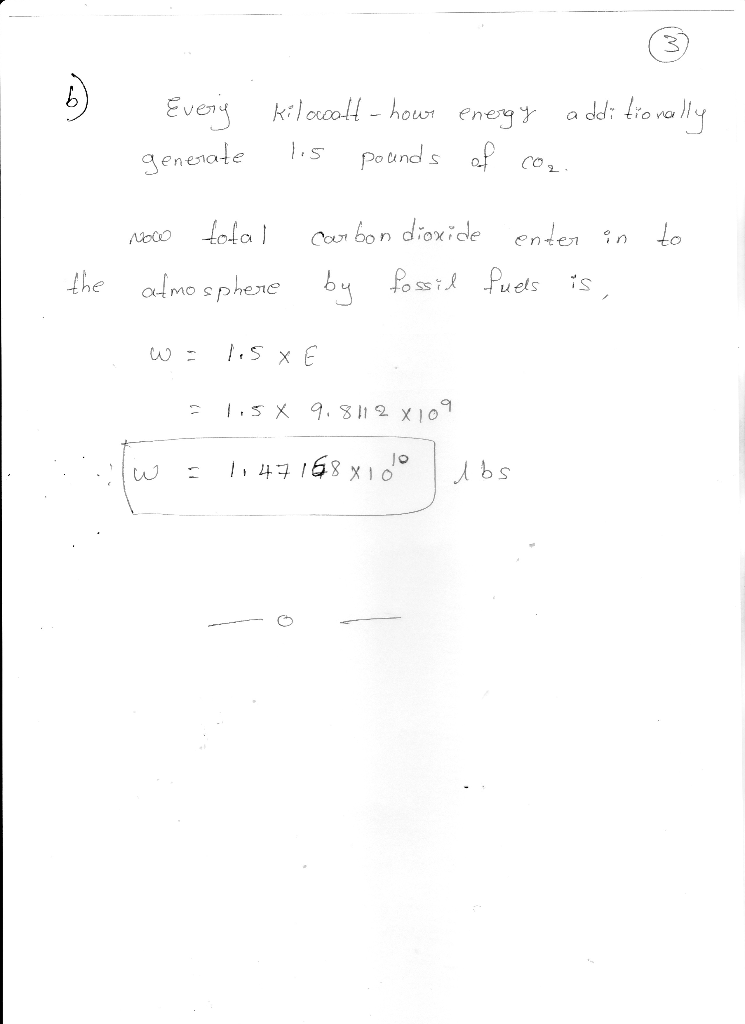Homework Help Question & Answers

A certain region currently has wind tarms capable ot generating a total ot 2800 megawatts (2 8 gigawatts) of power Complete parts (a) and (b) belouw อ. Assuming wind arms ypical y genera e 40 o her c...Add Answer of: A certain region currently has wind tarms capable ot generating a total ot 2800 megawatts (2 8 gigawatts) of power Complete parts (a) and (b) belouw อ. Assuming wind arms ypical y genera e 40 o her c...
More Homework Help Questions Additional questions in this topic.

• 3. Use the probability generating function Px)(s) to find (a) E[X(10)] (b) VarX(10)] (c) P(X(5)-2) . ( 4.2 Probability Generating Functions The probability generating function (PGF) is a us...

Need Online Homework Help?

Get FREE EXPERT Answers
WITHIN MINUTES
Related Questions